2017-07-08 00:47:35 vonuo 阅读数 14805
• ###### Python-数据库

Python数据库编程视频教程，数据库是MySQL，讲解Python链接MySQL数据库，并对数据库进行增删改查操作。

10902 人正在学习 去看看 尹成

GDAL是空间数据处理的开源包，支持多种数据格式的读写。遥感图像是一种带大地坐标的栅格数据，遥感图像的栅格模型包含以下两部分的内容：

GDAL读写遥感数据的代码：

``````from osgeo import gdal
import os

class GRID:

#读图像文件
dataset=gdal.Open(filename)       #打开文件

im_width = dataset.RasterXSize    #栅格矩阵的列数
im_height = dataset.RasterYSize   #栅格矩阵的行数

im_geotrans = dataset.GetGeoTransform()  #仿射矩阵
im_proj = dataset.GetProjection() #地图投影信息

del dataset
return im_proj,im_geotrans,im_data

#写文件，以写成tif为例
def write_img(self,filename,im_proj,im_geotrans,im_data):
#gdal数据类型包括
#gdal.GDT_Byte,
#gdal .GDT_UInt16, gdal.GDT_Int16, gdal.GDT_UInt32, gdal.GDT_Int32,
#gdal.GDT_Float32, gdal.GDT_Float64

#判断栅格数据的数据类型
if 'int8' in im_data.dtype.name:
datatype = gdal.GDT_Byte
elif 'int16' in im_data.dtype.name:
datatype = gdal.GDT_UInt16
else:
datatype = gdal.GDT_Float32

#判读数组维数
if len(im_data.shape) == 3:
im_bands, im_height, im_width = im_data.shape
else:
im_bands, (im_height, im_width) = 1,im_data.shape

#创建文件
driver = gdal.GetDriverByName("GTiff")            #数据类型必须有，因为要计算需要多大内存空间
dataset = driver.Create(filename, im_width, im_height, im_bands, datatype)

dataset.SetGeoTransform(im_geotrans)              #写入仿射变换参数
dataset.SetProjection(im_proj)                    #写入投影

if im_bands == 1:
dataset.GetRasterBand(1).WriteArray(im_data)  #写入数组数据
else:
for i in range(im_bands):
dataset.GetRasterBand(i+1).WriteArray(im_data[i])

del dataset

if __name__ == "__main__":
os.chdir(r'D:\Python_Practice')                        #切换路径到待处理图像所在文件夹
run = GRID()
print proj
print geotrans
print data
print data.shape
run.write_img('LC81230402013164LGN00_Rewrite.tif',proj,geotrans,data) #写数据``````

``````import numpy as np
data = data.astype(np.float)
ndvi = (data-data)/(data+data)                         #3为近红外波段；2为红波段
run.write_img('LC81230402013164LGN00_ndvi.tif',proj,geotrans,ndvi) #写为ndvi图像``````

python大数据处理面经 相关内容

2017-05-02 08:09:00 weixin_34119545 阅读数 11
• ###### Python-数据库

Python数据库编程视频教程，数据库是MySQL，讲解Python链接MySQL数据库，并对数据库进行增删改查操作。

10902 人正在学习 去看看 尹成

### 1.3　地理数据处理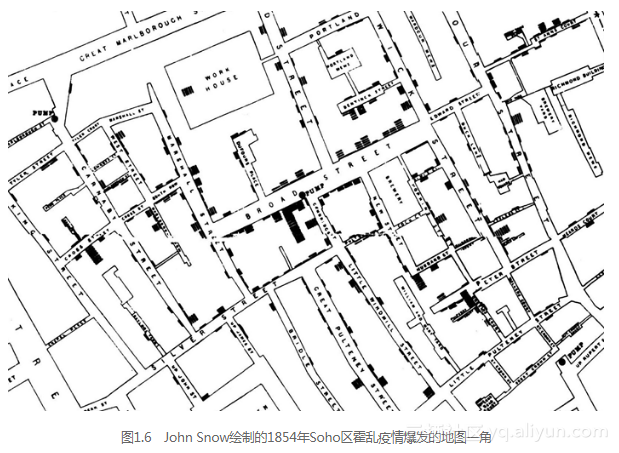python大数据处理面经 相关内容

2019-09-18 19:38:24 awpc21 阅读数 256
• ###### Python-数据库

Python数据库编程视频教程，数据库是MySQL，讲解Python链接MySQL数据库，并对数据库进行增删改查操作。

10902 人正在学习 去看看 尹成

### 对使用Python的相关职位做一个总结

#### python职业成长路径：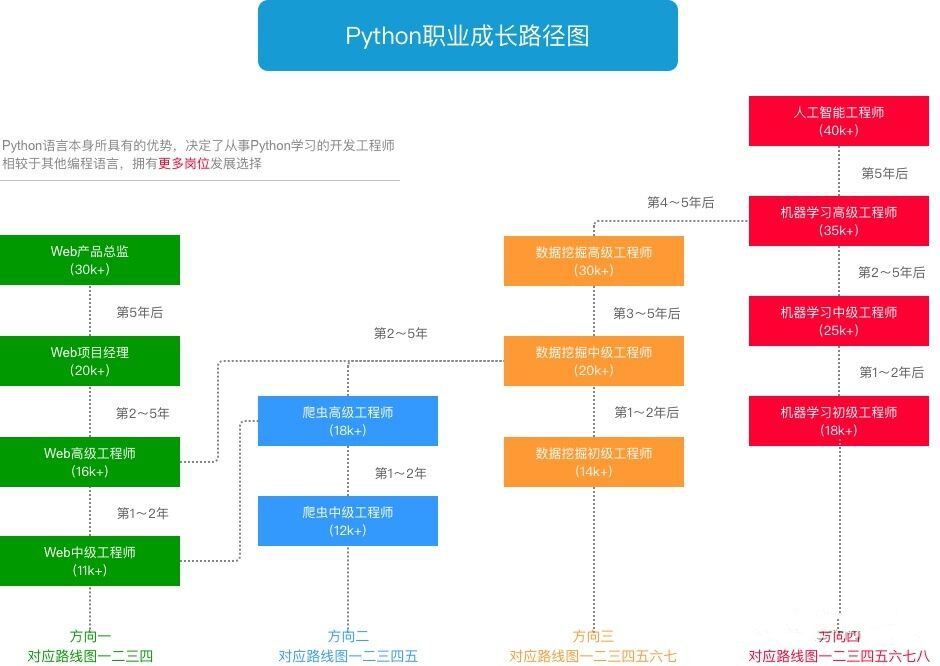#### Python职业要求：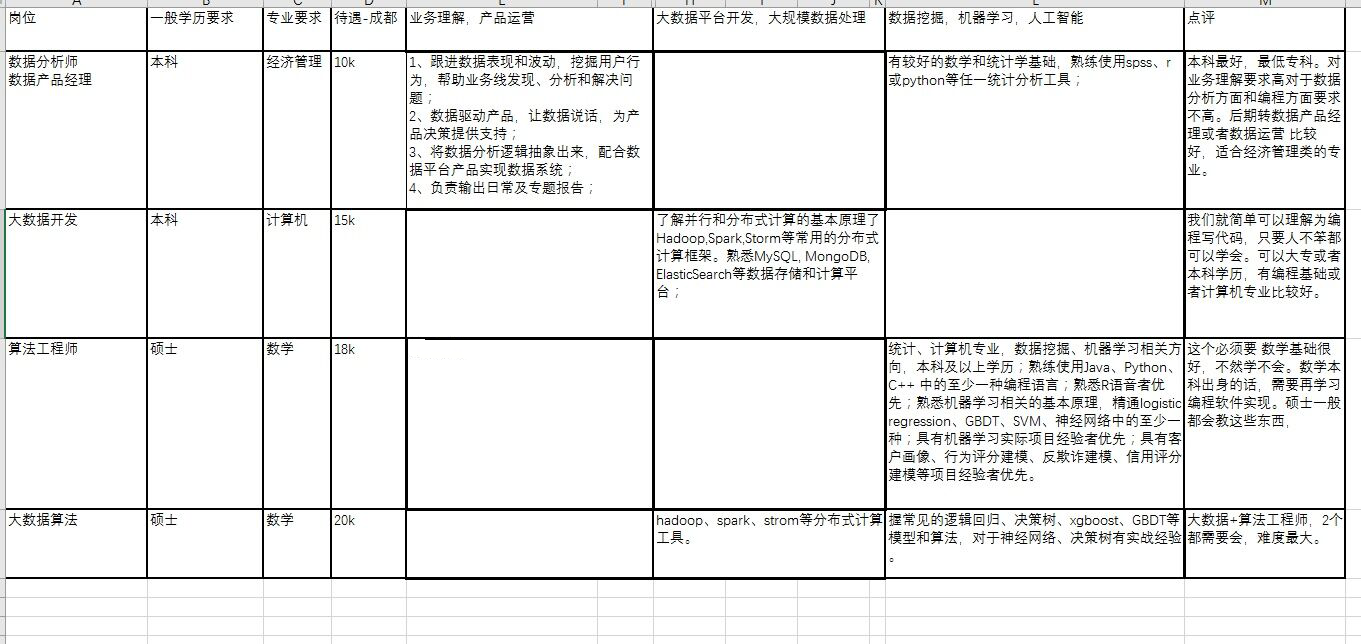#### 岗位要求：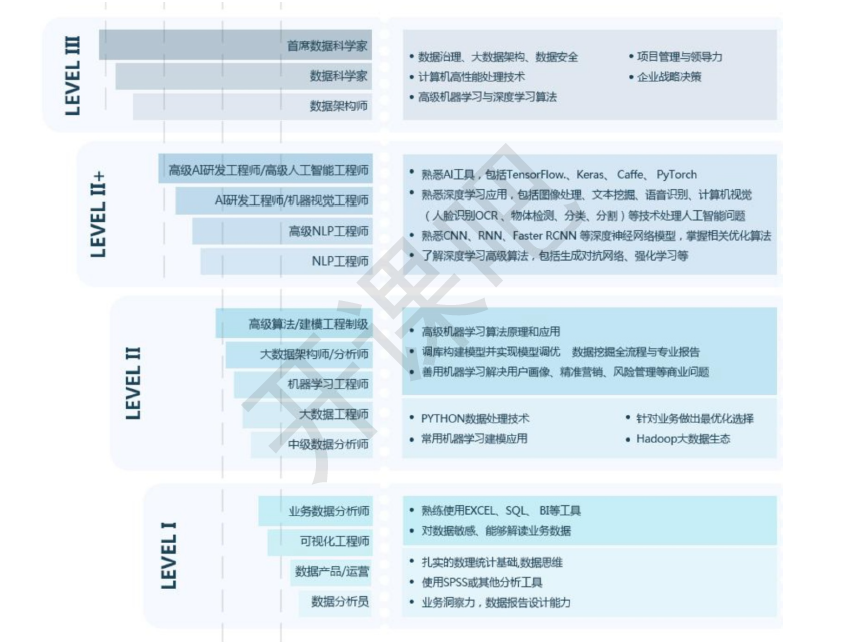#### 数据领域技能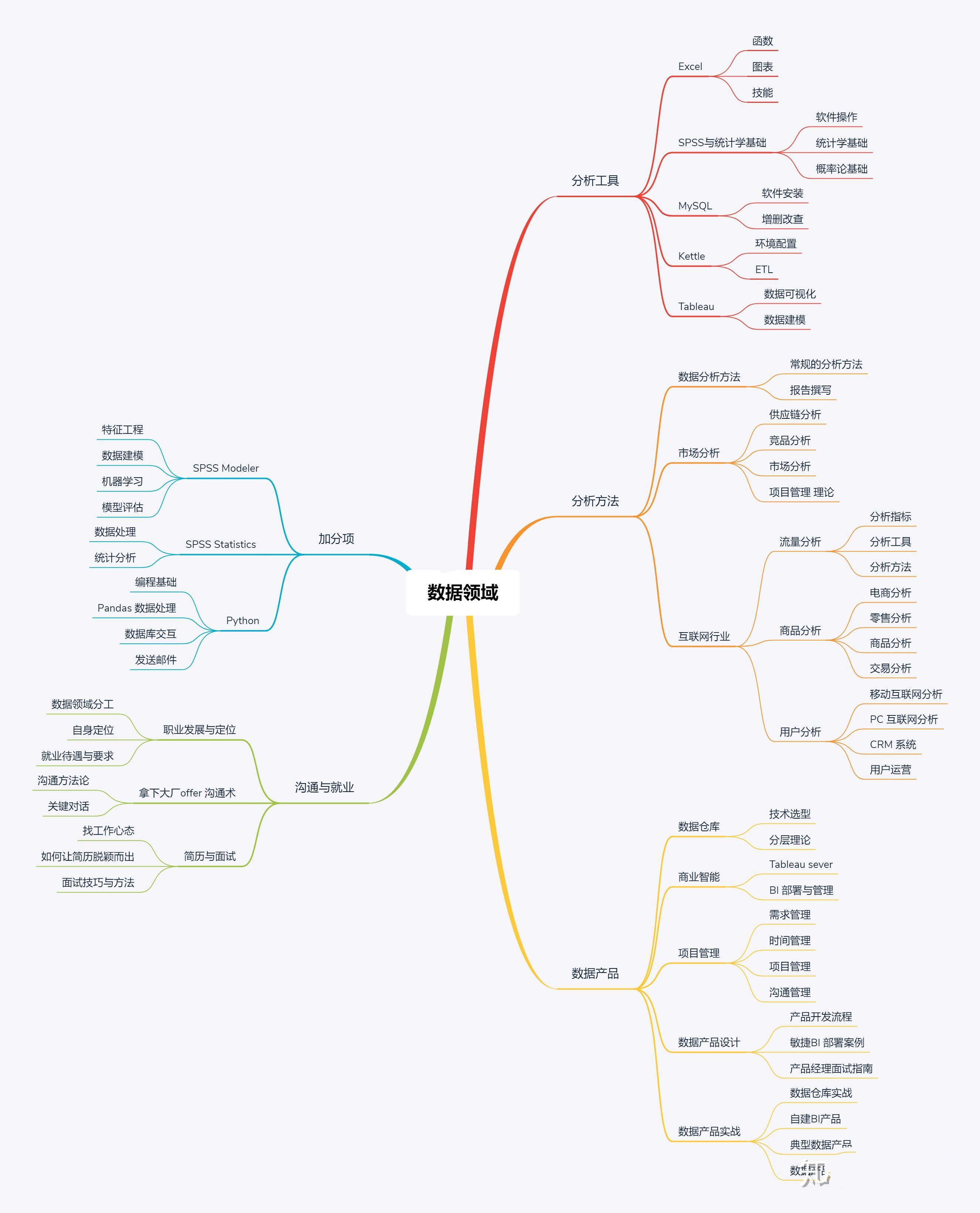python大数据处理面经 相关内容

2018-10-22 12:48:54 Stephen_shijun 阅读数 3792
• ###### Python-数据库

Python数据库编程视频教程，数据库是MySQL，讲解Python链接MySQL数据库，并对数据库进行增删改查操作。

10902 人正在学习 去看看 尹成

1.环境

python版本：python3.6.1

2.numpy库产生随机数几种方法

``````import numpy as np
``````
numpy.random
 rand(d0, d1, ..., dn) In : x=np.random.rand(2,5) In : x Out: array([[ 0.84286554,  0.50007593,  0.66500549,  0.97387807,  0.03993009],        [ 0.46391661,  0.50717355,  0.21527461,  0.92692517,  0.2567891 ]]) randn(d0, d1, ..., dn)查询结果为标准正态分布 In : x=np.random.randn(2,5) In : x Out: array([[-0.77195196,  0.26651203, -0.35045793, -0.0210377 ,  0.89749635],        [-0.20229338,  1.44852833, -0.10858996, -1.65034606, -0.39793635]]) randint(low,high,size) 生成low到high之间（半开区间 [low, high)），size个数据 In : x=np.random.randint(1,8,4) In : x Out: array([4, 4, 2, 7]) random_integers(low,high,size) 生成low到high之间（闭区间 [low, high)），size个数据 In : x=np.random.random_integers(2,10,5) In : x Out: array([7, 4, 5, 4, 2])

3.散点图

```x x轴
y y轴
s   圆点面积
c   颜色
marker  圆点形状
alpha   圆点透明度                #其他图也类似这种配置```
``````N=50
# height=np.random.randint(150,180,20)
# weight=np.random.randint(80,150,20)
x=np.random.randn(N)
y=np.random.randn(N)
plt.scatter(x,y,s=50,c='r',marker='o',alpha=0.5)
plt.show()``````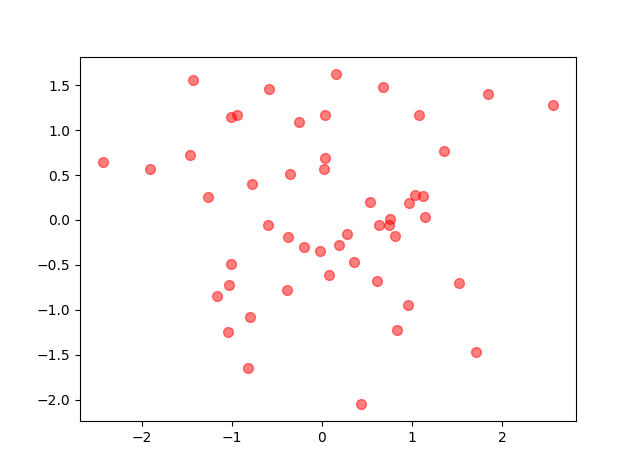4.折线图

``````x=np.linspace(-10000,10000,100) #将-10到10等区间分成100份
y=x**2+x**3+x**7
plt.plot(x,y)
plt.show()``````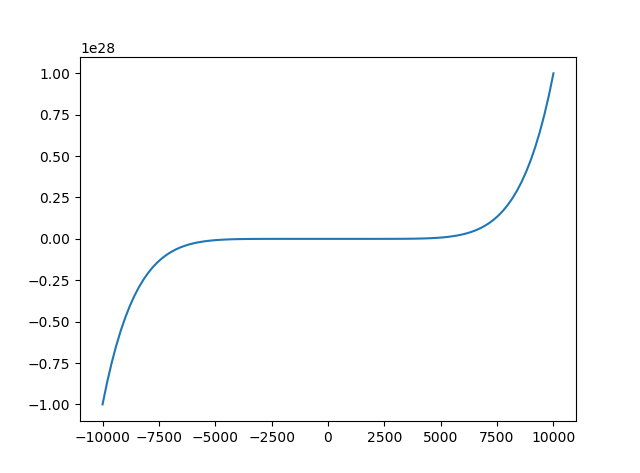5.条形图

``````N=5
y=[20,10,30,25,15]
y1=np.random.randint(10,50,5)
x=np.random.randint(10,1000,N)
index=np.arange(N)
plt.bar(left=index,height=y,color='red',width=0.3)
plt.bar(left=index+0.3,height=y1,color='black',width=0.3)
plt.show()``````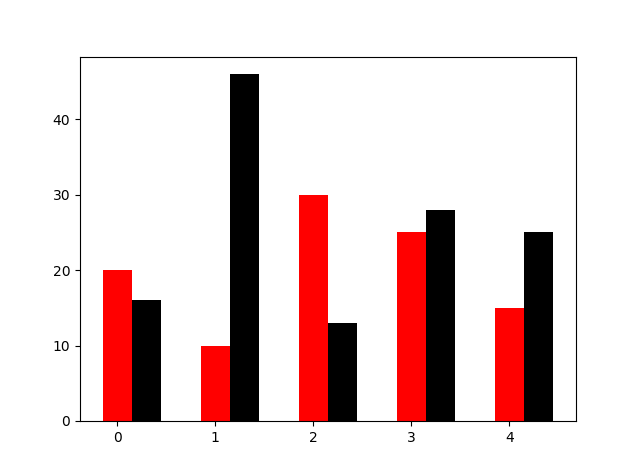orientation设置横向条形图

``````N=5
y=[20,10,30,25,15]
y1=np.random.randint(10,50,5)
x=np.random.randint(10,1000,N)
index=np.arange(N)
# plt.bar(left=index,height=y,color='red',width=0.3)
# plt.bar(left=index+0.3,height=y1,color='black',width=0.3)
#plt.barh() 加了h就是横向的条形图，不用设置orientation
plt.bar(left=0,bottom=index,width=y,color='red',height=0.5,orientation='horizontal')
plt.show()``````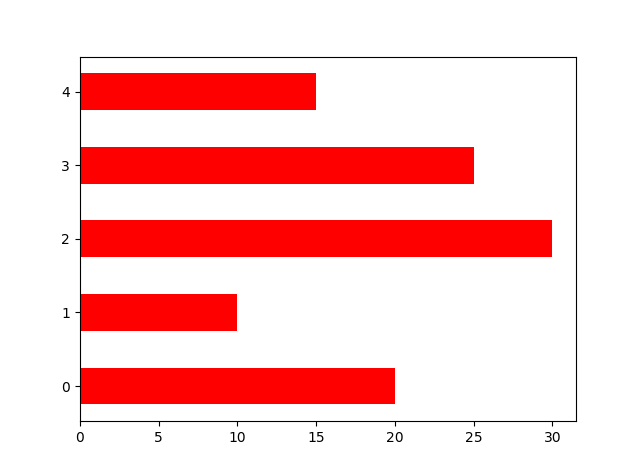6.直方图

``````m1=100
sigma=20
x=m1+sigma*np.random.randn(2000)
plt.hist(x,bins=50,color="green",normed=True)
plt.show()``````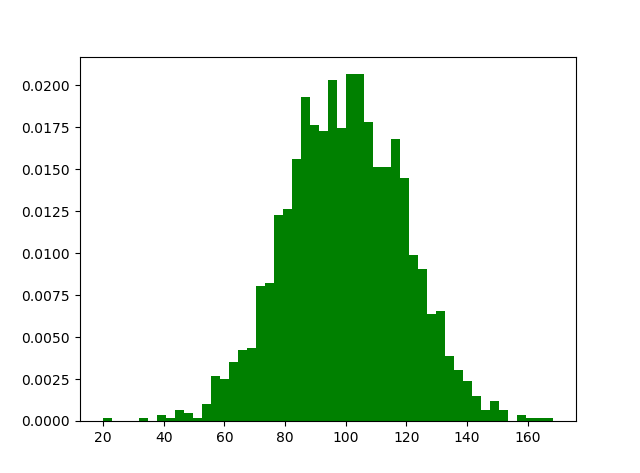```# #双变量的直方图
# #颜色越深频率越高
# #研究双变量的联合分布```
``````#双变量的直方图
#颜色越深频率越高
#研究双变量的联合分布
x=np.random.rand(1000)+2
y=np.random.rand(1000)+3
plt.hist2d(x,y,bins=40)
plt.show()
``````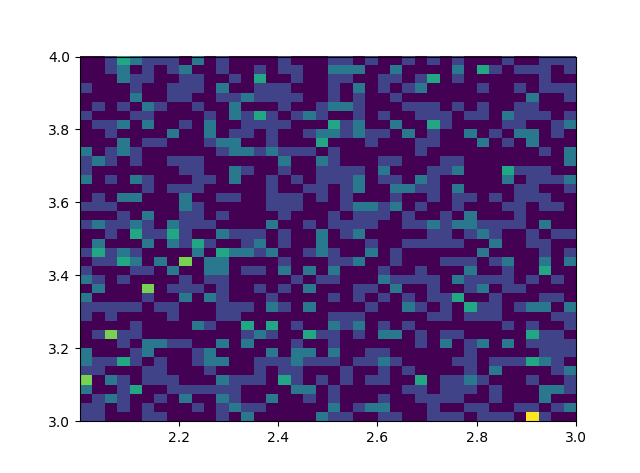7.饼状图

`#设置x,y轴比例为1：1，从而达到一个正的圆`
`#labels标签参数,x是对应的数据列表,autopct显示每一个区域占的比例,explode突出显示某一块,shadow阴影`
``````labes=['A','B','C','D']
fracs=[15,30,45,10]
explode=[0,0.1,0.05,0]
#设置x,y轴比例为1：1，从而达到一个正的圆
plt.axes(aspect=1)
plt.show()``````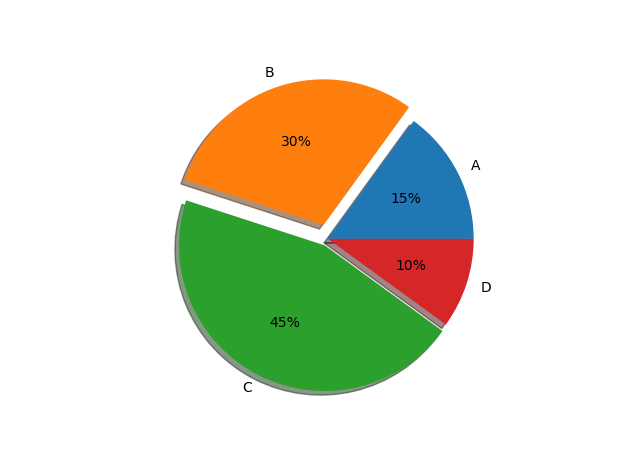8.箱型图

``````import matplotlib.pyplot as plt
import numpy as np
data=np.random.normal(loc=0,scale=1,size=1000)
#sym 点的形状，whis虚线的长度
plt.boxplot(data,sym="o",whis=1.5)
plt.show()
``````
`#sym 点的形状，whis虚线的长度`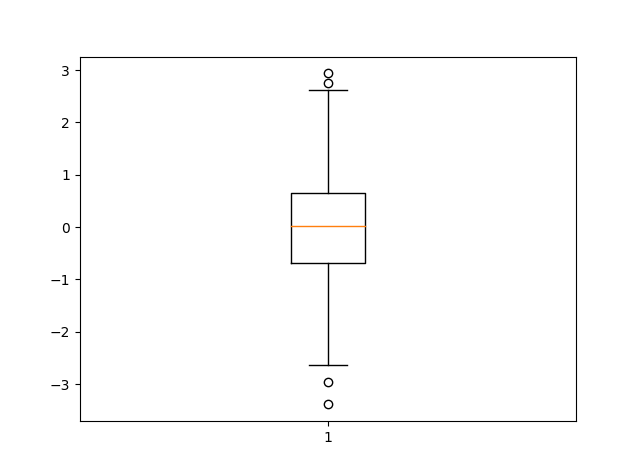python大数据处理面经 相关内容

2004-11-23 13:08:00 naive1010 阅读数 2271
• ###### Python-数据库

Python数据库编程视频教程，数据库是MySQL，讲解Python链接MySQL数据库，并对数据库进行增删改查操作。

10902 人正在学习 去看看 尹成

Python 是由 Guido van Rossum 开发的、可免费获得的、非常高级的解释型语言。其语法简单易懂，而其面向对象的语义功能强大（但又灵活）。Python 可以广泛使用并具有高度的可移植性。

 ```>>> s = "mary had a little lamb" >>> s # index is zero-based 'm' >>> s = 'x' # changing element in-place fails Traceback (innermost last): File "", line 1, in ? TypeError: object doesn't support item assignment >>> s[11:18] # 'slice' a subsequence 'little ' >>> s[:4] # empty slice-begin assumes zero 'mary' >>> s # index 4 is not included in slice [:4] ' ' >>> s[5:-5] # can use "from end" index with negatives 'had a little' >>> s[:5]+s[5:] # slice-begin & slice-end are complimentary 'mary had a little lamb' ```

in 关键字

 ```>>> s = "mary had a little lamb" >>> for c in s[11:18]: print c, # print each char in slice ... l i t t l e >>> if 'x' in s: print 'got x' # test for char occurrence ... >>> if 'y' in s: print 'got y' # test for char occurrence ... got y ```

 ```>>> s2 = """Mary had a little lamb ... its fleece was white as snow ... and everywhere that Mary went ... the lamb was sure to go""" >>> print s2 Mary had a little lamb its fleece was white as snow and everywhere that Mary went the lamb was sure to go ```

 ```>>> s3 = "this /n and /n that" >>> print s3 this and that >>> s4 = r"this /n and /n that" >>> print s4 this /n and /n that ```

 ``` fh = open('c://autoexec.bat') for line in fh.readlines(): print line ```

cStringIO 模块

 ```>>> import cStringIO >>> fh = cStringIO.StringIO() >>> fh.write("mary had a little lamb") >>> fh.getvalue() 'mary had a little lamb' >>> fh.seek(5) >>> fh.write('ATE') >>> fh.getvalue() 'mary ATE a little lamb' ```

string 模块也许是 Python 1.5.* 标准发行版中最常用的模块。实际上，在 Python 1.6 或更高版本中，string 模块中的功能将作为内置字符串方法（在撰写本文时，详细信息尚未发布）。当然，任何执行文本处理任务的程序也许应该用以下这行开头：

 ``` import string ```

string 模块包含了几种类型的事物，如函数、方法和类；它还包含了公共常量的字符串。例如：

string 用法例 1

 ```>>> import string >>> string.whitespace '/011/012/013/014/015 ' >>> string.uppercase 'ABCDEFGHIJKLMNOPQRSTUVWXYZ' ```

string 还包括了以常见方式（可以结合这些方式来构成几种罕见的转换）转换字符串的函数。例如：

string 用法例 2

 ```>>> import string >>> s = "mary had a little lamb" >>> string.capwords(s) 'Mary Had A Little Lamb' >>> string.replace(s, 'little', 'ferocious') 'mary had a ferocious lamb' ```

string 用法例 3

 ```>>> import string >>> s = "mary had a little lamb" >>> string.find(s, 'had')5>>> string.count(s, 'a')4 ```

string 用法例 4

 ```>>> import string>>> s = "mary had a little lamb" >>> L = string.split(s) >>> L ['mary', 'had', 'a', 'little', 'lamb'] >>> string.join(L, "-") 'mary-had-a-little-lamb' ```

re 模块废弃了在老的 Python 代码中使用的 regexregsub 模块。虽然相对于 regex 仍然有几个有限的优点，不过这些优点微不足道，不值得在新代码中使用。过时的模块可能会从未来的 Python 发行版中删除，并且 1.6 版可能有一个改进的接口兼容的 re 模块。所以，规则表达式仍将使用 re 模块。

 ```>>> import re >>> s = "mary had a little lamb" >>> if re.search("m", s): print "Match!" # char literal Match! >>> if re.search("[@A-Z]", s): print "Match!" # char class ... # match either at-sign or capital letter ... >>> if re.search("/d", s): print "Match!" # digits class ... ```

 ```ABC([d-w]*/d/d?)+XYZ ```

 ```ABC1234567890XYZ ABCd12e1f37g3XYZ ABC1XYZ ```

 ```ABC123456789dXYZ ABCdefghijklmnopqrstuvwXYZ ABcd12e1f37g3XYZ ABC12345%67890XYZ ABCD12E1F37G3XYZ ```

python大数据处理面经 相关内容